LANGUAGE
•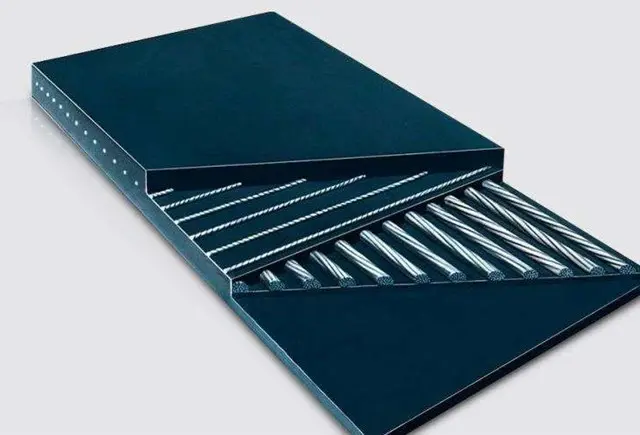•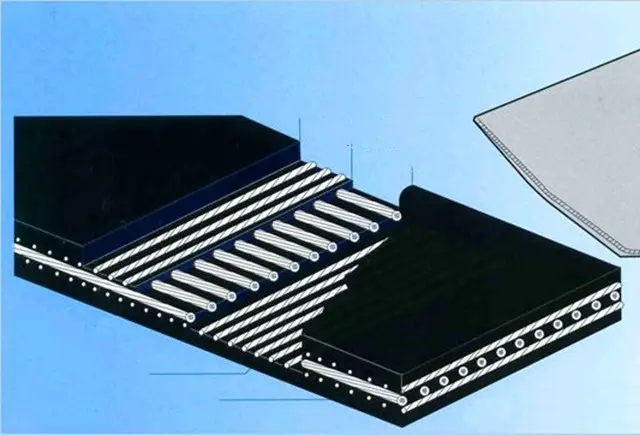•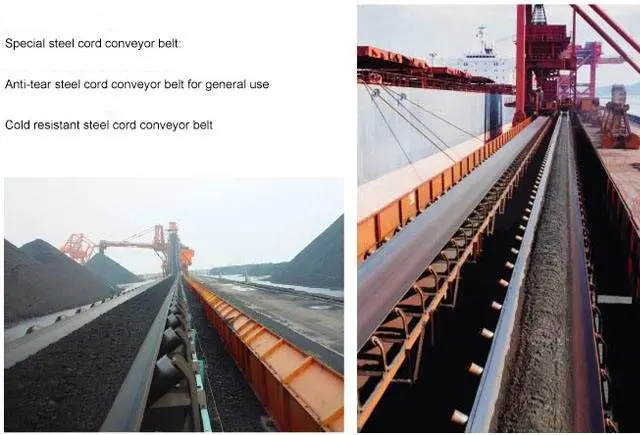•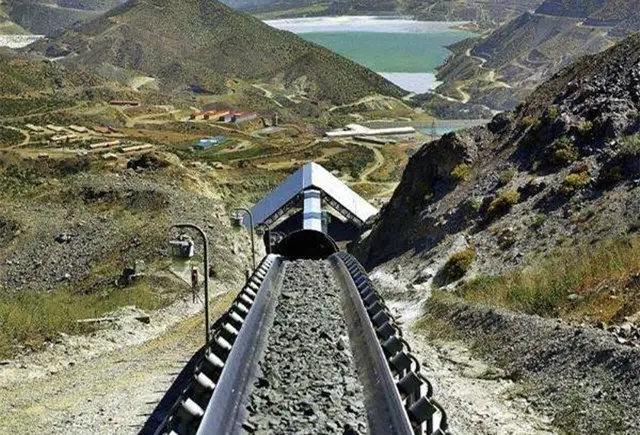•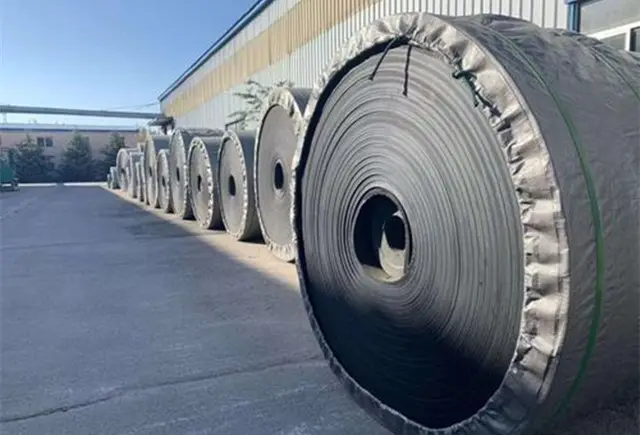# Steel Cord Conveyor Belt

Steel cord conveyor belts have proved to be a very reliable and cost-efficient mode of bulk material handling in long distance conveyors. SKE provide transverse reinforcementand, embedded sensor coil and normal type steel cord belt conveyor belt.

• Applications
• Datasheet
• Related
Applications

Steel cord conveyor belt (steel wire rope core conveyor belt) is extensively applied in coal mines, Lignite mines, Aluminium mines, Copper mines, ports, metallurgy, power stations, steel industries, Ore Handling Plants, Cement Industries, chemical sectors for long-distance, large amount and high-speed conveying of materials.

The steel cord conveyor belt can be divided into ordinary type, flame-retardant type, heat-resistant type, wear-resistant type, cold resistant type, acid and alkali resistant type, oil resistant type, etc. according to the covering rubber performance. According to its composition, we can divide it into normal type, transverse reinforcement, and sensor line loop embedded steel wire core conveyor belt.

1. High Tensile Strength: steel cord conveyor belt is suitable for transferring materials with long-distance, high intensity and large load.
2. Small Elongation In Use: a very short take-up stroke distance needed.
3. High Adhesion: the steel cord is coated with zinc on the surface, highly adhered to the special core rubber to resistant to heavy impact.
4. Good Trough Ability: as the belt body has no transverse carcass, it is easy to form a deep trough to load more materials without a leak.
5. Smaller Diameter of Driving Pulley: the belt body has a layer of longitudinally arranged steel cords as its carcass to resistant to flex fatigue. Therefore, driving pulley with a smaller diameter can be used to reduce energy consumption.
6. Even Tension of Steel Cords: thanks to our advanced techniques in manufacturing, the steel cords are very evenly arranged with the same tension, so the belts are well balanced in running and difficult to run away.

Tech Datasheet
 Type Data Thickness St500 Operation Tension(N/mm) = 75Standard diameter (mm) = 2.7Max. diameter(mm) = 3.0Min. Cord strength(kN) = 6.9Cord pitch(mm) = 12.0 4/4 St630 Operation Tension(N/mm) = 90Standard diameter (mm) = 2.8Max. diameter(mm) = 3.0Min. Cord strength(kN) = 6.9Cord pitch(mm) = 10.0 4/4 St800 Operation Tension(N/mm) = 110Standard diameter (mm) = 3.1Max. diameter(mm) = 3.5Min. Cord strength(kN) = 8.8Cord pitch(mm) = 10.0 4/4 St1000 Operation Tension(N/mm) = 140Standard diameter (mm) = 3.7Max. diameter(mm) = 4.0Min. Cord strength(kN) = 13.2Cord pitch(mm) = 12.0 5/5 St1250 Operation Tension(N/mm) = 175Standard diameter (mm) = 4.2Max. diameter(mm) = 4.5Min. Cord strength(kN) = 16.5Cord pitch(mm) = 12.0 5/5 St1400 Operation Tension(N/mm) = 200Standard diameter (mm) = 4.4Max. diameter(mm) = 4.8Min. Cord strength(kN) = 18.5Cord pitch(mm) = 12.0 6/6 St1600 Operation Tension(N/mm) = 225Standard diameter (mm) = 4.7Max. diameter(mm) = 5.0Min. Cord strength(kN) = 21.1Cord pitch(mm) = 12.0 8/6 St1800 Operation Tension(N/mm) = 255Standard diameter (mm) = 5.1Max. diameter(mm) = 5.5Min. Cord strength(kN) = 24.2Cord pitch(mm) = 12.0 8/6 St2000 Operation Tension(N/mm) = 28.5Standard diameter (mm) = 5.5Max. diameter(mm) = 6.0Min. Cord strength(kN) = 26.4Cord pitch(mm) = 12.0 8/6 St2250 Operation Tension(N/mm) = 320Standard diameter (mm) = 5.7Max. diameter(mm) = 6.2Min. Cord strength(kN) = 30.0Cord pitch(mm) = 12.0 8/6 St2500 Operation Tension(N/mm) = 355Standard diameter (mm) = 6.8Max. diameter(mm) = 7.2Min. Cord strength(kN) = 41.2Cord pitch(mm) = 15.0 8/6 St2800 Operation Tension(N/mm) = 400Standard diameter (mm) = 7.3Max. diameter(mm) = 7.8Min. Cord strength(kN) = 46.0Cord pitch(mm) = 15.0 8/6 St3150 Operation Tension(N/mm) = 450Standard diameter (mm) = 7.6Max. diameter(mm) = 8.1Min. Cord strength(kN) = 51.9Cord pitch(mm) = 15.0 8/8 St3500 Operation Tension(N/mm) = 570Standard diameter (mm) = 8.0Max. diameter(mm) = 8.6Min. Cord strength(kN) = 57.1Cord pitch(mm) = 15.0 8/8 St4000 Operation Tension(N/mm) = 570Standard diameter (mm) = 8.6Max. diameter(mm) = 8.9Min. Cord strength(kN) = 66.0Cord pitch(mm) = 15.0 8/8 St4500 Operation Tension(N/mm) = 645Standard diameter (mm) = 9.1Max. diameter(mm) = 9.7Min. Cord strength(kN) = 79.2Cord pitch(mm) =  16.0 8/8 St5000 Operation Tension(N/mm) = 715Standard diameter (mm) = 10.5Max. diameter(mm) = 10.9Min. Cord strength(kN) = 93.5Cord pitch(mm) = 17.0 8.5/8.5 St5400 Operation Tension(N/mm) = 760Standard diameter (mm) = 10.8Max. diameter(mm) = 11.3Min. Cord strength(kN) = 101.0Cord pitch(mm) = 17 9/9 St5800 Operation Tension(N/mm) = 830Standard diameter (mm) = 11.5Max. diameter(mm) = 11.8Min. Cord strength(kN) = 109.0Cord pitch(mm) = 17 10/10 St6300 Operation Tension(N/mm) = 900Standard diameter (mm) = 12.0Max. diameter(mm) = 12.3Min. Cord strength(kN) = 118.0Cord pitch(mm) = 17 12/10 St6700 Operation Tension(N/mm) = 950Standard diameter (mm) = 12.7Max. diameter(mm) = 13.0Min. Cord strength(kN) = 133.0Cord pitch(mm) = 18.0 12/10

 Wear Resistant Data Characteristics X Min.Tension N/mm =25Max. Abrasion mm³=120 Heavy-duty cover for coarse and sharp materialResistant against impacts and cuts.Complies with: X (DIN), RMA1, H (ISO), M (AS), M24 (BS) Y Min.Tension N/mm =20Max. Abrasion mm³=150 Wear-resistant cover for normal operating conditionsComplies with: Y (DIN), RMA 1&2, L (ISO), N (AS), N17 (BS) W Min.Tension N/mm =18Max. Abrasion mm³=90 Cover with excellent resistance against erosive wearFor small-sized, abrasive materialComplies with: W (DIN), RMA 1&2, D (ISO)

 Flame Resistant Data Characteristics K Min.Tension N/mm =20Max. Abrasion mm³=200 Abrasion-resistant cover with flame-resistant characteristicsaccording to ISO 340 VT Min.Tension N/mm =17Max. Abrasion mm³=175 Cover with fire-resistant properties according to DIN

 Heat Resistant Data Characteristics T120 Min.Tension N/mm =15Max. Abrasion mm³=175 Heat-resistant, abrasion-resistant cover for temperatures up to 120 °C

Contact Now

Top